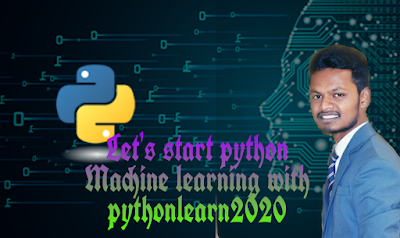## Machine Learning:

Welcome to python Machine Learning section , This is second part of my  blog post. In this crash course we will cover the following point:

### Syllabus for the Machine Learning:

1] Linear Regression with python
2] Logistic Regression in python
3] K- Nearest Neighbors with python
4] Decision Trees and Random Forest with python
5] Support Vector Machine
6] K- Mean Clustering
7] Principle Component Analysis
8] Recommend System
9] Natural Language Processingfig 01) Machine Learning tutorial-algorithms-course-projects

### Some Introduction of Syllabus Topic:

1. Linear Regression with python:
Linear Regression is machine learning algorithm based on supervised learning. It performs a different regression task.
2. Logistic Regression in python:
Logistic regression is also supervised learning classification algorithm used to predict the probability of a target variable.
3.  K- Nearest Neighbors with python:
The K-nearest neighbors is a type of supervised machine learning algorithms. KNN is algorithm used for complex classification.
4. Support Vector Machine:
In machine learning, support-vector machine are supervised models with associated learning algorithm that analyze data used for classification
5. K- Mean Clustering:
K-means clustering is simplest and popular unsupervised machine learning algorithms. it's also used for clustering classification.
6.  Principle Component Analysis:
Principal components analysis is a technique used to emphasize variation and bring out strong pattern in a dataset. It's often used to make data easy to explore and visualized.(PCA)
7. Recommend System:
Recommend Systems is a subclass of information filtering system that seeks to predict the "rating" or preference a user would give to an item. They are primarily used in commercial applications.
8. Natural Language Processing:
Natural language processing involves the reading and understanding of spoken and written language through the medium of a computers.
(Note: If you like my blog post then please comment and share)
Tags:Machine Learning tutorial-algorithms-course-projects-beginners
BEST OF LUCK!!!!!
Previous
Next Post »

### comparison python vs r vs sas vs spss

python  vs r vs sas vs spss : Welcome everyone, Today we will see comparison between  r vs python vs sas vs spss . So Let's start : ...